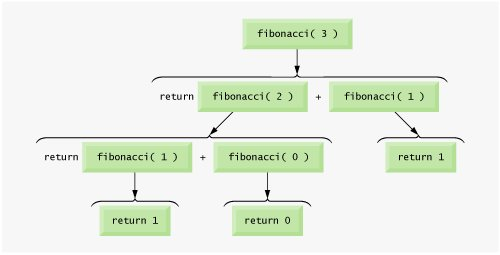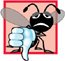Example Using Recursion: Fibonacci Series

Example Using Recursion Fibonacci Series

The Fibonacci series

0, 1, 1, 2, 3, 5, 8, 13, 21, ...

begins with 0 and 1 and has the property that each subsequent Fibonacci number is the sum of the previous two Fibonacci numbers.

The series occurs in nature and, in particular, describes a form of spiral. The ratio of successive Fibonacci numbers converges on a constant value of 1.618.... This number, too, frequently occurs in nature and has been called the golden ratio or the golden mean. Humans tend to find the golden mean aesthetically pleasing. Architects often design windows, rooms and buildings whose length and width are in the ratio of the golden mean. Postcards are often designed with a golden mean length/width ratio.

The Fibonacci series can be defined recursively as follows:

fibonacci(0) = 0

fibonacci(1) = 1

fibonacci(n) = fibonacci(n 1) + fibonacci(n 2)

The program of Fig. 6.30 calculates the nth Fibonacci number recursively by using function fibonacci. Notice that Fibonacci numbers also tend to become large quickly, although slower than factorials do. Therefore, we chose the data type unsigned long for the parameter type and the return type in function fibonacci. Figure 6.30 shows the execution of the program, which displays the Fibonacci values for several numbers.

Figure 6.30. Demonstrating function fibonacci.

(This item is displayed on page 293 in the print version)

1 // Fig. 6.30: fig06_30.cpp
2 // Testing the recursive fibonacci function.
3 #include
4 using std::cout;
5 using std::cin;
6 using std::endl;
7
8 unsigned long fibonacci( unsigned long ); // function prototype
9
10 int main()
11 {
12 // calculate the fibonacci values of 0 through 10
13 for ( int counter = 0; counter <= 10; counter++ )
14 cout << "fibonacci( " << counter << " ) = "
15 << fibonacci( counter ) << endl;
16
17 // display higher fibonacci values
18 cout << "fibonacci( 20 ) = " << fibonacci( 20 ) << endl;
19 cout << "fibonacci( 30 ) = " << fibonacci( 30 ) << endl;
20 cout << "fibonacci( 35 ) = " << fibonacci( 35 ) << endl;
21 return 0; // indicates successful termination
22 } // end main
23
24 // recursive method fibonacci
25 unsigned long fibonacci( unsigned long number )
26 {
27  if ( ( number == 0 ) || ( number == 1 ) ) // base cases
28  return number;
29  else // recursion step
30  return fibonacci( number - 1 ) + fibonacci( number - 2 );
31 } // end function fibonacci

 fibonacci( 0 ) = 0 fibonacci( 1 ) = 1 fibonacci( 2 ) = 1 fibonacci( 3 ) = 2 fibonacci( 4 ) = 3 fibonacci( 5 ) = 5 fibonacci( 6 ) = 8 fibonacci( 7 ) = 13 fibonacci( 8 ) = 21 fibonacci( 9 ) = 34 fibonacci( 10 ) = 55 fibonacci( 20 ) = 6765 fibonacci( 30 ) = 832040 fibonacci( 35 ) = 9227465

The application begins with a for statement that calculates and displays the Fibonacci values for the integers 010 and is followed by three calls to calculate the Fibonacci values of the integers 20, 30 and 35 (lines 1820). The calls to fibonacci (lines 15, 18, 19 and 20) from main are not recursive calls, but the calls from line 30 of fibonacci are recursive. Each time the program invokes fibonacci (lines 2531), the function immediately tests the base case to determine whether number is equal to 0 or 1 (line 27). If this is true, line 28 returns number. Interestingly, if number is greater than 1, the recursion step (line 30) generates two recursive calls, each for a slightly smaller problem than the original call to fibonacci. Figure 6.31 shows how function fibonacci would evaluate fibonacci( 3 ).

Figure 6.31. Set of recursive calls to function fibonacci.

(This item is displayed on page 294 in the print version)This figure raises some interesting issues about the order in which C++ compilers will evaluate the operands of operators. This is a separate issue from the order in which operators are applied to their operands, namely, the order dictated by the rules of operator precedence and associativity. Figure 6.31 shows that evaluating fibonacci( 3 ) causes two recursive calls, namely, fibonacci( 2 ) and fibonacci( 1 ). But in what order are these calls made?

Most programmers simply assume that the operands are evaluated left to right. The C++ language does not specify the order in which the operands of most operators (including +) are to be evaluated. Therefore, the programmer must make no assumption about the order in which these calls execute. The calls could in fact execute fibonacci( 2 ) first, then fibonacci( 1 ), or they could execute in the reverse order: fibonacci( 1 ), then fibonacci( 2 ). In this program and in most others, it turns out that the final result would be the same. However, in some programs the evaluation of an operand can have side effects (changes to data values) that could affect the final result of the expression.

The C++ language specifies the order of evaluation of the operands of only four operatorsnamely, &&, ||, the comma (,) operator and ?:. The first three are binary operators whose two operands are guaranteed to be evaluated left to right. The last operator is C++'s only ternary operator. Its leftmost operand is always evaluated first; if it evaluates to nonzero (true), the middle operand evaluates next and the last operand is ignored; if the leftmost operand evaluates to zero (false), the third operand evaluates next and the middle operand is ignored.

Common Programming Error 6.25Writing programs that depend on the order of evaluation of the operands of operators other than &&, ||, ?: and the comma (,) operator can lead to logic errors.

Portability Tip 6.3Programs that depend on the order of evaluation of the operands of operators other than &&, ||, ?: and the comma (,) operator can function differently on systems with different compilers.

A word of caution is in order about recursive programs like the one we use here to generate Fibonacci numbers. Each level of recursion in function fibonacci has a doubling effect on the number of function calls; i.e., the number of recursive calls that are required to calculate the nth Fibonacci number is on the order of 2n. This rapidly gets out of hand. Calculating only the 20th Fibonacci number would require on the order of 220 or about a million calls, calculating the 30th Fibonacci number would require on the order of 230 or about a billion calls, and so on. Computer scientists refer to this as exponential complexity. Problems of this nature humble even the world's most powerful computers! Complexity issues in general, and exponential complexity in particular, are discussed in detail in the upper-level computer science course generally called "Algorithms."

Performance Tip 6.8Avoid Fibonacci-style recursive programs that result in an exponential "explosion" of calls.C++ How to Program (5th Edition)
ISBN: 0131857576
EAN: 2147483647
Year: 2004
Pages: 627

Similar book on Amazon